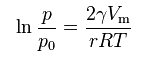# Calculating change in vapour pressure using Kelvin Equation

This CalcTown calculator calculats the change in vapour pressure  due to a curved liquid/vapor interface (meniscus) using kelvin equation.

K
mL
mm
N/m

#### ResultFor water, molar volume is generally taken to be 18 mL , while for mercury, it is taken to be 14.8 mL